A spreadsheet is a visual matrix of functional dependencies. A cell may contain data or a function definition. S, S+, and S- are K implementations of essential, extended, and minimal spreadsheet functionality.

## Behavior

By default, the S spreadsheet has 100 rows and 26 columns, labelled a through z:A spreadsheet instance can be dynamically configured to have n rows and m columns, labelled C through C[m-1], by assigning the variables N and C in the instance directory:

```.S.N:10
.S.C:`first`second`third
```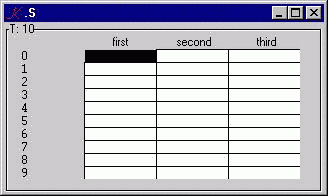We want b0 to be a0+1 and c0 to be a0+b0. So, into a0, enter 12; into b0, enter =a0+1; into c0, enter =a0+b0:The foreground of a0 is black. b0 and c0 are blue, to indicate calculated values.

A spreadsheet keeps track of which quantities need to recalculate themselves when updates occur. For example, if we update a0, we should see b0 and c0 immediately recalculate: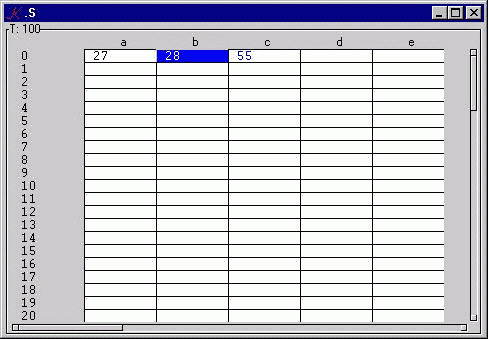If we now type 13 into b0 (a formula cell), we see that c0 recalculates, but b0 presents itself in red, to indicate that its value may be inconsistent, and needs to be recalculated: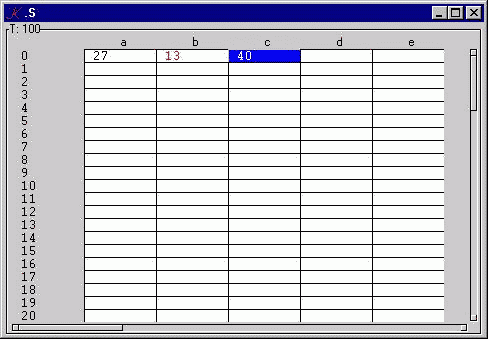For example, if we now type 14 into a0, b0 and c0 recalculate: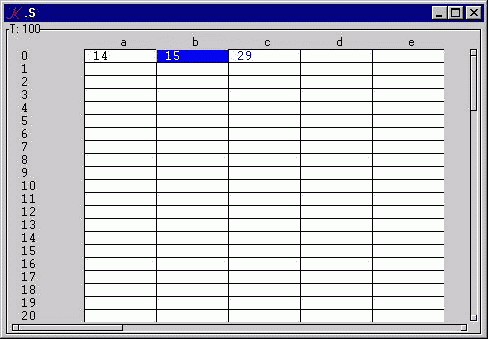To clear a cell, type just the equality symbol. For example, type = into c0 to expunge the formula a0+b0. This severs the dependence of c0 on a0 and b0: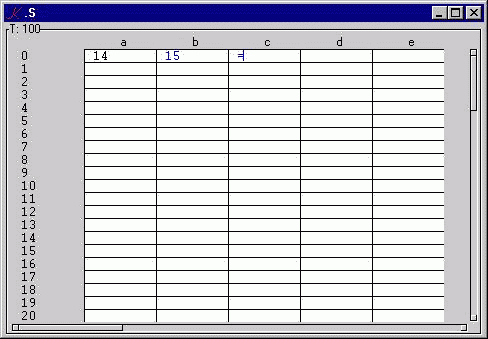Vector expressions can be used in formulas. For example, enter a series of values into the a column, and then, in a2, enter the formula =+/a: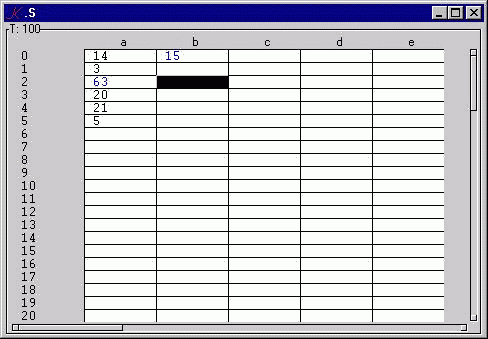The formula is interpreted to mean: a0+a1+a3+...+a99. Observe that a2 knows to exclude itself from the set of summand cells.

Each spreadsheet cell knows how to evaluate K. For example, we can directly enter a K expression into a cell and have it execute, leaving behind its result as the cell value: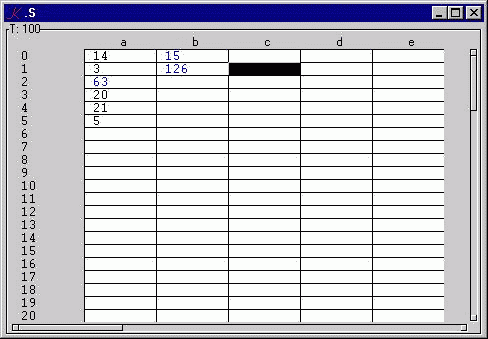In our basic spreadsheet model, a label is a string: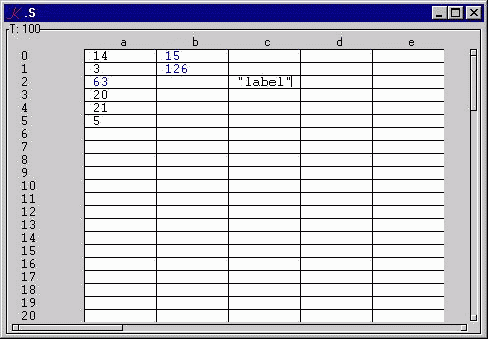Labels are "inert". They are rendered using a gray background.

A formula loop is trapped and signalled. For example, if we attempt to define a0 as b0-1, a "cycle!" error will be signalled to the spreadsheet: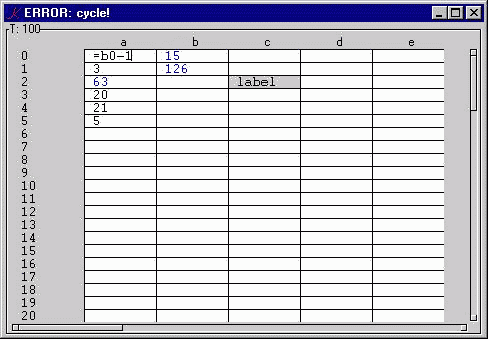Press the escape key to restore the previous value and clear the error.

A formula may consist of any valid K expression, including arbitrary functions and variables. Names beginning with a dot are evaluated over the K tree.

For example,

```.my.fun:{x+y*z}
```

Now, in cell b4, enter:

```=.my.fun[a0;a1;a2]
```

which produces:## Logic

A spreadsheet is an instance of the variable .S containing a table T with columns C and rows !N. T[c;r] is the value of cell cr, for c in C and r in !N. The value of the empty cell is 0.

A cell can be associated with a formula which computes its value. The formula is stored apart from the value, in the formula table X. The executable representation of the formula is stored in Y. Two tables Z and ZZ contain lists of cells derived from the formulas in X. Thus, Z[c;r] is a list of cells used by X[c;r]; that is, the cells on which T[c;r] directly depends. ZZ[c;r] contains a list of cells which directly use T[c;r]; that is, the cells whose formulas contain occurrences of cr.

X, Y, Z and ZZ are modified when a formula is entered into a cell. The formula is parsed, and information derived during parsing is stored in Y, Z, and ZZ. The formula itself is stored in X.

When an update is applied to cell cr, the spreadsheet is selectively invalidated. Selective invalidation marks only those cells which depend on the updated cell. ZZ[c;r] is processed recursively until cells are reached on which no cells depend. After all cells which depend on cr have been marked invalid, all marked cells are recalculated and restored to the valid state.

cr is invalidated by appending (c;r) to V, the list of invalid cells. cr is validated in two steps: first, replace T[c;r] with the result of executing Y[c;r], and then remove (c;r) from V.

When T[c;r] is cleared (by entering a naked equality symbol), the corresponding cells of the formula and dependency tables X, Y, and Z are cleared, and (c;r) is removed from each of the lists Z ./:Z[c;r]. That is, if T[c;r] depends on ds, then (c;r) is a member of ZZ[d;s]. In order to clear T[c;r], (c;r) must be removed from ZZ[d;s], the list of cells which depend on ds.

T[c;r] is displayed in one of three colors: black, if it is not a formula-cell; blue, if it is a formula-cell and is consistent with the cells on which it depends; and red, if it is a formula-cell and is not known to be consistent.

When T[c;r] is updated from the screen, the input string either begins with = or it does not. If the string begins with =, then either a formula is being defined or a cell is being cleared; in each case, W is set to (), the empty list. If the string does not begin with =, then a value is being entered, and W is set to (c;r). Thus, W records whether the last update was a formula definition (or clear) or a value update. If cr, the cell to be displayed, has no formula, then W is irrelevant: draw cr black. If cr has a formula, then draw it red if W~(c;r), else draw it blue.

The processing cycle:

Suppose there is screen input to T[c;r]. K calls the S parser T.c..g on the input value, which is always a string. The result of T.c..g is then assigned to T[c;r]. The S trigger T..t fires. Invalidation occurs, followed by validation. When T..t is finished executing, every cell in T is valid. Those cells in T which have changed are re-drawn to the screen. Each cell-draw involves a pair of K function-calls: one to T..f, to format the cell-value as a string, and one to T..fg, to draw the cell-foreground in the appropriate color.

## Analysis

S consists of two parts: .s, the directory of functions and data shared by all spreadsheet instances, and .S, the prototype spreadsheet instance, or "template". If a single, stand-alone instance of S is required, then .S itself will do. If many spreadsheets are needed, or the spreadsheet variable is to be part of some larger screen structure, then copies of .S can be made using K assignment:

```new:.S
new.:.S.
```

.S.T is a K table: a directory of equal-length lists. K programs can update spreadsheet cells by assigning values to variables in T. In this, the basic model, assignment is limited to cellular, that is atomic update. This restriction is lifted in S+ by extending .S.T..t, the trigger on T.

The heart of parsing in S consists of translating expressions over cell-names -- "formulas" -- into executable K. The rules are simple:

```cr -> .s.get[_d;(c;r)]
c  -> if ~c=*K .s.get[_d;c] else (.s.get[_d]'(..;(c;i);..)) where ~i=*|K
```

The complicated business in the second rule ensures that a column can contain its own aggregation. For example, if T[`b;5] is defined to be +/a, then +/ is translated by the if-clause of the second rule, since *K is `b, not `a. But if T[`a;5] is defined to be +/a, then *K is `a, and the else clause of the rule is used, since we want to exclude T[`a;5] from the sum over column a.

Unless otherwise noted, x is the path to the spreadsheet instance, and y is a cell-index. For example, functions called under an update .S..T[`xyz;17] will see `.S for x, and (`xyz;17) for y. In what follows, I will often refer to T[`xyz;17] as y.

## Analytical documentation

```\d .s

Function context:  there is only ever one copy of this

A:(_ci 97+!26;_ci 48+!10)

*A defines the alphabet over which column names can be defined
*|A is a digit list

set:{:[eq1[x;y;z," "];def[x;y;1_ z];_n~r:. z;0;r]}

z: string to validate/parse

if z is a formula, define y
else if r, the execution of z, is nil, return 0
else return r

eq1:{.[x;`W;:;:[r:"="=*z;();y]];r}

set W to () if z is a formula, else to y;
return 1 if z is a formula, else 0

def:{:[""~z;clr[x;y;x .`Z,y];prs[x;y;z]];.[x;`V;,;,y];0}

z: string to define

if z is empty, clear y, else parse z;
invalidate y;
return 0

Observe that the value of an empty cell is 0.  This allows us to
define number-valued formulas over cells without values.  Also note
that formula-cells are empty until they have been validated.

clr:{(`X`Y`Z,\:y).[x;;:;]'(;;());{.[x;`ZZ,z;_dv;y]}[;y]/[x;z]}

z: cells on which y depends

empty X, Y, and Z at positions corresponding to y;
for each cell z[i] on which y depends, remove y from ZZ of z[i]

prs:{(`X`Y,\:y).[x;;:;]'(z;,/spl[x;y]'(&1>':z _lin"._`\"",*A)_ z)}

z: string to parse

save z in X;
save the raze of the split of each col-partition of z in Y

A col-partitioning of a string cuts the string at each initial
col-name character.  A col-name character is in the alphabet
*A, extended by "._`" and the quotation mark "\"".  For
example, the col-name partitions of "abc10+.myfoo `xyz" are
("abc10+";".myfoo ";"`xyz").  Calculating which partitions
begins with a cell-name is deletgated to spl.

spl:{:[~(*z)_in*A;z;,/@[(0,(z _lin,/A)?0)_ z;0;nam[x;y]]]}

z: col partition

if z does not begin with a character in *A, return z
else cut z into cell name and a remainder;
apply nam[x;y] to the cell name, raze and return the result

nam:{:[""~*|r:(0,(z _lin*|A)?1)_ z;vec[x;y;*r];sca[x;y;r]]}

z: cell name

if the row-part of r is empty
then generate the expression for getting all of the column
else generate the expression for getting a cell

sca:{".s.get[_d;",(5:dep[x;y;z]),"]"}

z: ("col";"row")

return a string which, when executed, returns the value of T[col;row]
call dep first, to see if the formula cycles

e.g.: the formula a12-3 is parsed ".s.get[_d;(`a12)]-3"

vec:{"(.s.get[_d]'",(5:dep[x;y]'(z{(x;y)}/:\$!x`N)_dv\$y),")"}

z: "col"

return a string which, when executed, returns the value of T[col];
fabricate a cell reference for every row in T;
call dep on each cell to see if the formula creates cycles

e.g.: the formula +/a is parsed ".s.get[_d]'P", where P is
((`a;0);...;(`a;N-1))_dv y; i.e., the get of each cell except
y, the cell defined by the formula

dep:{cyc[x;y;r:(`;0)\$z];.[x;`Z,y;,;,r];.[x;`ZZ,r;,;,y];r}

z: ("col";"row")

check for cycles on r, which (`col;row);
if there are none, record the dependency of y on r in Z and ZZ;
return r

cyc:{if[y _in(,z),don[x;x .`Z,z];'"cycle!"]}

z: (`col;row)

signal an error if y is x or in the list of cells on which z depends

don:{:[()~y;y;y,,/x _f/:x{x .`Z,y}/:y]}

z:((c;r);...;(c;r)

return the list of cells on which z depends

act:{inv[x;y]'x .`ZZ,y;x get/:x`V}

y: cell which has been assigned

invalidate every cell which depends on y;
validate every invalid cell

inv:{if[~z _in y,x`V;.[x;`V;,;,z];_f[x;y,,z]'x .`ZZ,z]}

z: list of cells which depend on y

if z is valid:
invalidate z
recurse, invalidating every cell which depends on z

get:{:[~y _in _dv/x`V`W;x .`T,y;dat[x;y]]}

if y is valid, return its value (from T)
else calculate its value

dat:{r::[@x .`X,y;x .`T,y;. x .`Y,y];.[x;`T,y;:;r];.[x;`V;_dv;y];r}

if y is not a formula, then r is T at y
else y is the result of evaluating the formula for y;
update T at y with r;
remove y from the list of invalid cells
return r

fd0:{,(`N_;!x;.+(`e`f`l;(0;-8\$;"")))}

x: N

generate N_ column, with attributes e, f, l

fds:{(y;x#0;.+(`f`g`fg`bg;`.s.f`.s.g`.s.fg`.s.bg))}

x: N
y: column symbol

generate a data column of 0's, with attributes f, g, fg, bg

f:{:[x~0;8#" ";\$x]}

format: if x is 0, 8 blanks, else default format

g:{set[dr _d;cl[_v;_i];x]}

validate: update this cell in the current instance with x

fg:{:[@(d:dr _d).`X,i:cl[_v;_i];0;i~d`W;990000;99]}

foreground color:

if this cell in the current instance is not a formula, black
else if this formula-cell has been updated manually, red
else blue

bg:{:[-3=4:x;808080]}

background color:

if this cell in the current instance is a string, gray

cl:{(`\$1_*|(&"."=v)_ v:\$x;*y)}

x: `. ... instance.table.color
y: index into x

return canonical cell id (`col;row).

e.g., if x is `.S.T.b and y is ,3 then return (`b;3)

dr:{`\$(- 1+(|v)?".")_ v:\$x}

x: `. ...instance.table

return instance `. ... instance.

e.g., if x is `.S.T then return `.S

\d .S

.S is a typical spreadsheet instance, or template.
New instances can be defined through simple assignment:

new:.S
new.:.S

C:`\$/:*.s.A

the symbols `a`b`c ... to be used as column names in T

N:100

the number of rows in T

T..d:"..s.fd0[N],N .s.fds/:C"

T depends on C and N.  T has 1+#C columns, each of which has
N rows.  Each column of T is a list-variable.  The initial
column is N_, which exists for the sole purpose of enumerating
the rows.  This column is treated as pure decoration:  input is
not allowed, and the label is turned off.  For each of the
remaining columns, four attributes are defined:  a format
function f, a validation function g, a foreground color function
fg, and a background color function bg.  The functions fd0 and
fds generate, respectively, the row enumeration column N_ and
the data columns C.

T..t:".s.act[_d;_i];"

an update to any variable in T will cause .s.act to be called.

X..d:Y..d:".+(C;(#C)#,N#_n)"
Z..d:ZZ..d:".+(C;(#C)#,N#,())"

The four variables X, Y, Z, and ZZ are defined as tables which
are structurally identical to T.  X- and Y- cells are initialized
to _n, to simplify testing for formula-cellishness.  Z- and ZZ-
cells are initialized to (), since the cells of these tables
are cell-lists.

V..d:W..d:"C;N;()"

The two variables V and W are initialized to ().  V is a cell-
list; W is a cell.

\d ~

the attribute dictionary of .S

c:`form

.S is a form

a:`T

.S's arrangement is the table T

x:95
y:30
.S's initial size

```

## Extensions: S+

This section describes the S+ spreadsheet, which extends the core model S by implementing the following ten features:

• Defined display: the user can specify the format function of a cell
• Defined foreground: the user can specify the foreground color of a cell
• Defined background: the user can specify the background color of a cell
• Defined edit: the user can specify whether a cell is editable
• Defined conversion: the user can specify the conversion function of a cell
• Attributes can be replicated and cleared
• Row- and column-ranges can be specified in a formula
• Formulas can be viewed and edited through an alternate input field
• Recalculation of cells can be immediate or postponed
• All forms of assignment to T are supported

In S, there is one command letter, =, which defines (or clears) a formula. In S+, the command letters are = (or C), D, E, F, B, and G. A command is either a command letter or a command letter followed by either a column name or a row number. An empty command is the instruction to clear the corresponding attribute. For example,

```=       Clear the current cell formula

D9      Clear the formats in all cells from here to row 9
```

### Defined display, editing, foreground,background colors, conversion

```D 7.3\$                              display width 7, 3 digits of precision

G {:[0<r:. x;r;'"must be >0"]}      convert if >0 else signal error

E.1                                 editable

F 9900                              foreground color = green

B {:[x<0;.red;.blue]}               background = red if negative, blue if not
```

### Replication

Attributes (D,E,F,B,G) are absolute. A replicated attribute has the same meaning in every cell in which it appears: green is the same green everywhere. The reference of a formula can be absolute or relative. For example:

```=c a1+2                 replicate a1+2 from here to column c

=c \$1 +2                replicate a1+2, b1+2, c1+2 from here to column c
```

If the current column is a and the subsequent columns are b and c, then the second formula -- a formula-scheme -- will produce three distinct formulas: a1+2 in the current cell, b1+2 in the b cell, and c1+2 in the c cell. The meaning of a formula-scheme is location-dependent. The dollar sign is schematic for the name of the current column, or in the case of row-wise replication, for the current row:

```=12 a\$+3                replicate a\$+3 from here to row 12
```

Attributes can also be replicated:

```F17 99                  blue foreground from here to row
```

Finally, values can be replicated (as formulas without cell-dependencies):

```=d 17                   replicate 17 from here to column d
```

### Row- and column-ranges

Vector operations in the basic model apply only over whole columns. For example,

```= +/a                   sum of a
```

To define the current cell as the sum of a0 through a5 times the sum of a6 through a12:

```= (+/a0:a5)*+/a6:a12    product of sums of row-ranges
```

: means "to". Alternatively,

```= (+/a0:d0)*+/a0:k0     products of sums of column ranges
```

Ranges can be used within formula schemes. For example, if the current cell is a0, then:

```=5 +/b\$:k\$              +/b0:k0, +/b1:k1, ... ,+/b5:k5
```

The translations are extended to handle ranges:

```cr:cs -> (.s.get[_d]'((c;r);...;(c;s)))
cr:dr -> (.s.get[_d]'(c;r);...;(d;r)))
```

### Alternate input

An alternate-input field I has been provided for several reasons. First, the formula-definition of a cell can be displayed and edited. Second, the canonical form of a cell-value can be displayed using 5:. Third, label-valued cells can be edited (since they are always output-only in the extended version). Updates to the alternate input field are applied to the current cell, the index of which stored in the variable K.

### Recalculation

In the basic model, changing the value of a cell on which other cells depend causes the immediate recalculation of the dependent cells. There are circumstances in which immediate recalculation is expensive (if the calculations are costly) or misleading (if the intermediate states of the calculation are meaningless). Immediate recalculation also means that, if a spreadsheet is updated from a program source (e.g. a real-time feed), then it cannot be used interactively. A recalculation-checkbutton has therefore been added. With recalculation on, invalid cells are immediately validated. With recalculation off, the list of invalid cells accumulates until the switch is turned back on, at which point all invalid cells are recalculated. The state of being invalid-and-displayed (which cannot occur if calculation is on) is signalled by graying-out the background of the cell. Thus, for example, if c0=a0+b0, and recalculation is on, then an update to a0 will cause c0 to recalculate, as will an update to b0. With recalculation off, c0 will be added (once) to the invalid list, and updates to a0 and b0 will have no effect on c0's value until recalculation is turned back on.

### Assignment

In the basic model, the only form of programmatic assignment to T is cellular (atomic); for example, .T.a:101. In the extended model, the trigger function has been designed to detect and respond to any form of indexed assignment.

### Behavior

The extended model consists of a form .S with four components: the Calculate button, which controls recalculation; Q, the current contents of the selected cell; I, the alternate input field; and T, the spreadsheet itself.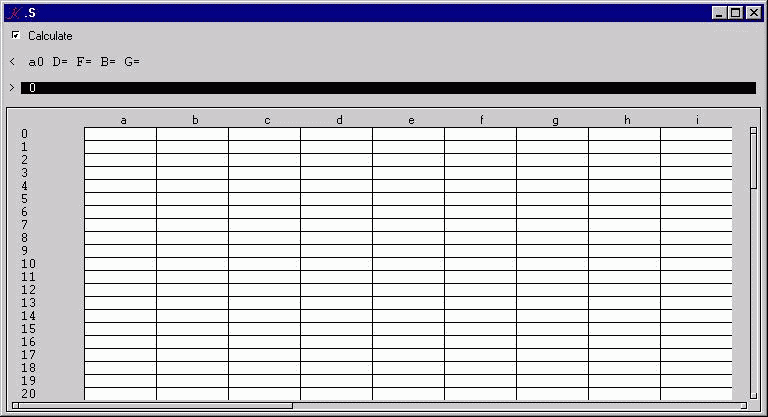Enter 1 to 20 into columns a0 to d4 programmatically:

```.S.T[`a`b`c`d;!5]:+1+5 4#!20
```Define column e as the row-sums, row 5 as the column-sums:

```in e0: =4 +/a\$:d\$
in a5: =e +/\$0:\$4
```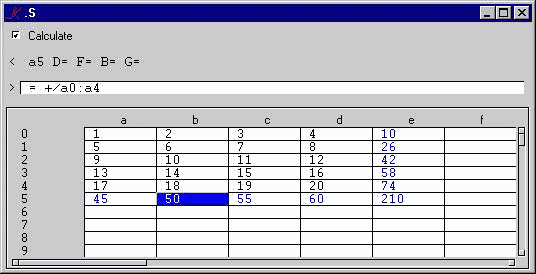Turn off Calculate, update a0 and c3; a5, c5, e0, e3, and e5 are invalid and pending.Turn Calculate back on; pending invalidities are validated:## Retractions: S-

The S- spreadsheet may be the minimal K implementation (Arthur Whitney, 1995).

The code verges on the trivial:

```S..t:".[`D;(;);{. y};S[]];S[.;`f]:9\$D[]"
S:D:.+(`a`b`c`d;4 6#,"")
```

(Foreground, label, and click attributes have been added to the version distributed with this paper.)Here's how it works:

Formulas are entered without a command letter, but in K notation. For example, the formula a0+b22 is defined: a+b.

Labels are entered as in S/S+, as strings: "abc".

Input is not processed; there is no parsing in S-.

S is a table of strings on the screen. D is a table of values.

For example, if a0 is 12 and b0 is the formula a+1, then S.a is "12" and S.b is "a+1", and D.a is 12 and D.b is 13.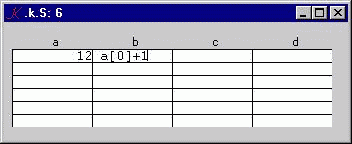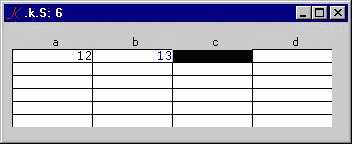and the value 2 is entered in S[`a;0]. There is no g function, so "2" is accepted and assigned to S[`a;0]. The trigger S..t fires and performs two pieces of work. First, S is re-evaluated, element-by-element, in the directory D, and the result of each evaluation is stored in the corresponding position of D. The value of D[`a;0] is now 2, and that of D[`b;0] is 3. Next, the f attribute of each variable in S is assigned the formatted values in D. After the trigger completes, the contents of f are written to the screen.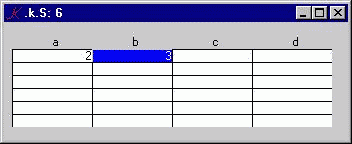## Operation

Copy the scripts for the three spreadsheet programs to a directory s.

In a command shell, type

`k s/s`

or

`k s/s_plus`

depending on whether you want to use the basic K spreadsheet S or the extended version S+.

Once in the K session, turn error-trapping on:

`\e 0`

Now make an instance of .S, the spreadsheet template:

```mysheet:.S
mysheet.:.S.```

````show\$`mysheet